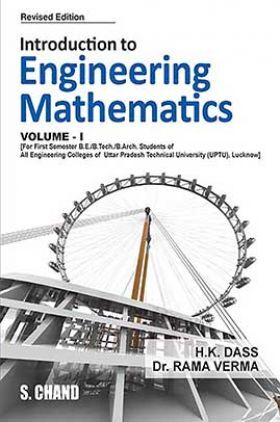# Introduction to Engineering Mathematics Vol-I

Sold ( 60 times )
38731 Views
MRP : ₹425.00
Price : ₹382.50
You will save : ₹42.50 after 10% Discount
Inclusive of all taxes

Save extra with 2 Offers

Get ₹ 50

Instant Cashback on the purchase of ₹ 400 or above

Product Specifications

 Publisher SChand Publications All Engineering Mathematics books by SChand Publications ISBN 9788121935241 Author: H K Dass Number of Pages 795 Available Available in all digital devicesAbout The Book Introduction to Engineering Mathematics Vol-I by H K Dass

Book Summary:

The book has been thoroughly revised according to the New Syllabi (2013-2014) of Gautam Buddha Technical University, Lucknow. Errors and misprints which came to my notice have been removed. Latest Solved Question Papers of 2010-2011 and 2012-2013 have been solved and included in the textbook.

Audience of the Book :

(FIRST SEMESTER)[For B.E./B.Tech./B.Arch. Students of First Semester of all Engineering Colleges of Gautam Buddha Technical University, Lucknow]

UNIT - I : DIFFERENTIAL CALCULUS – I

Leibnitz’s Theorem, Partial Derivatives, Euler’s theorem for homogeneous functions, Total derivatives,Change of Variables, Curve Tracing, Cartesian and Polar Coordinates.

UNIT - II : DIFFERENTIAL CALCULUS – II

Taylor’s and Maclaurin’s Theorems, Expansion of function of several variables, Jacobian, Approximation of Errors, Extrema of Functions of Several Variables, Lagrange’s Method of Multipliers (Simple Applications)

UNIT - III : LINEAR ALGEBRA

Inverse of a matrix by elementary transformations, Rank of a matrix (Echelon & Normal form), Linear Dependence, Consistency of Linear System of Equations and their Solution , Characteristic Equation, Eigen values and eigen vectors, Cayley-Hamilton Theorem, Application of matrices to engineering problems, A brief introduction to Vector Spaces, Subspaces, Rank & Nullity, Linear Transformations.

UNIT - IV : MULTIPLE INTEGRALS

Double and triple integrals, Change of order of integration, Change of variables, Application of integration to lengths, Volumes and Surface areas, Cartesian and Polar Coordinates, Beta and Gamma function,s Dirichlet’s integral and applications.

UNIT - V : VECTOR CALCULUS

Point function, Gradient, Divergence and curl and their physical interpretations, Vector identities, Directional derivatives, Line, Surface and volume Integrals, Applications of Green’s, Stoke’s and Gauss divergence theorems (without proofs).